# System of equations - 9th grade (14y) - math problems

#### Number of problems found: 584

• Two numbersThe sum of the two numbers is 1. Find both numbers if you know that half of the first is equal to one seventh of the second number.
• Two numbersThe difference between the two numbers is 74. If we divide a larger number by a smaller one, we get a quotient 7 and the rest of 2. Determine both numbers.
• Two numbers 7The sum of two consecutive even numbers is 30. Find the numbers.
• LegsCancer has 5 pairs of legs. The insect has 6 legs. 60 animals have a total of 500 legs. How much more are cancers than insects?
• YearsSusan is 3 years and as she will be old as Teodor now him will be 11. How old is Teodor today?
• Three numbers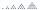What are three numbers that have the property: the sum of the first and second numbers' reciprocals is 12/7, the first and third 11/24, and the second and the third 3/8.
• Rabbits 3Viju has 40 chickens and rabbits. If in all there are 90 legs. How many rabbits are there with Viju?
• The difference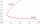The difference of two numbers is 1375. If their exact quotient is 12. Find the two numbers
• PoojaPooja and Deepa age is 4:5, 4 years back it was 8:11. What is the age of Pooja now?
• Three ints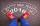The sum of three consecutive integers is 2016. What numbers are they?
• Arithmetic progression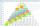In some AP applies: 5a2 + 7a5 = 90 s3 = 12 Find the first member a =? and difference d = ?
• Two numbersThe sum of two numbers is 1. Identify this two numbers if you know that the half of first is equal to the third of second number.
• Animals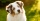All without two animals are dogs, all without two are parrots, all without two are cats, the others are chickens. How many animals of individual species does Ms. Novak take care of?
• The largerThe larger of two numbers is nine more than four times the smaller number. The sum of the two numbers is fifty-nine. Find the two numbers.
• Boxes200 boxes have been straightened in three rows. The first was 13 more than in the second, and in the second was one fifth more than in the third one. How many boxes are in each row?
• The sizeThe size of a Trapezium are 3/4×cm, ×cm 2(×+1)cm and 3(×+2)cm long respectively if it's perimeter is 60cm, calculate the length of each side.
• 3 masonsThree masons received 7,700 CZK. The second half received 1/2 more than the first and third twice more than the second mason. How much they each got crowns?
• Three figures - numbersThe sum of three numbers, if each is 10% larger than the previous one, is 662. Determine the figures.
• AntennasIf you give me two antennas will be same. If you give me again your two antenna I have a 5× so many than you. How many antennas have both mans?
• Saving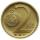Paul saves 155Kč in 46 2 Kc and 5Kc coins. How much saved 2Kc and 5Kc coins?

Do you have an interesting mathematical word problem that you can't solve it? Submit a math problem, and we can try to solve it.

We will send a solution to your e-mail address. Solved examples are also published here. Please enter the e-mail correctly and check whether you don't have a full mailbox.

Please do not submit problems from current active competitions such as Mathematical Olympiad, correspondence seminars etc...

Do you have a system of equations and looking for calculator system of linear equations? System of equations - math problems. Examples for 9th grade.